## Facebook Hacker Cup 2015: Qualification Round problem analysis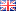Another year, another Facebook Hacker Cup, and another opportunity for smart people who know at least one programming language to put their problem solving skills to the test. As in earlier years, I will post a short analysis along with my solution for each problem, and I'll add some follow-up questions that people who have already solved the problems might find interesting.

#### A. Cooking the Books

Problem Statement (official link).

The qualification round starts off easily. For the first problem, we're given a number, and we're supposed to turn it into the highest/lowest value possible, by swapping just two digits. Warning: spoilers ahead!

Since the number is only (up to) 9 digits long, simply swapping all 9*8/2 = 36 pairs of digits and keeping track of the minimum/maximum obtained will do the trick. There is only one gotcha: you're not allowed to swap a zero into the leftmost position. Since this case is mentioned both in the text and in the sample data (10 cannot be turned into 01), this is unlikely to trip anyone up.

My solution in Python:
Python:
 ```1 2 3 4 5 6 7 8 9 10 11 12 13 14 ``` ```from sys import stdin T = int(stdin.readline()) for case in range(1, T + 1): digits = stdin.readline().strip() lo = hi = int(digits) for i in range(len(digits)): for j in range(len(digits)): t = list(digits) t[i],t[j] = t[j],t[i] # swap digits at index i and j if t != '0': n = int(''.join(t)) lo = min(lo, n) hi = max(hi, n) print('Case #%d: %d %d'%(case, lo, hi))```

Reader Challenge: if this is too simple for you (and let's be honest, it was pretty simple) then try to solve this variant: what if the numbers can be very large? Can you come up with a solution that works quickly for numbers of up to 100,000 digits?

#### B. New Year's Resolution

Problem Statement (official link).

The second problem is slightly more interesting. We are given a set of up to 20 pieces of food, each of which has some fixed mass of protein, carbohydrates and fat. We need to select a subset such that the sum of the protein, carbs and fat in the selection matches a given goal.

Like the previous problem, this one can easily be solved by brute force. For a set of N items, there are 2N possible subsets (including the set itself, and the empty set). Calculating the totals for a subset takes O(N) time (3 additions for each food item selected). Simply trying each subset yields an O(N 2N) algorithm, or about 20 million basic operations for N=20. With only 20 test cases, thats fast enough. The main challenge is to figure out a way to enumerate all subsets efficiently.

Since I'm old school, I used bitmasks in C:
C:
 ```1 2 3 4 5 6 7 8 9 10 11 12 13 14 15 16 17 18 19 20 21 22 23 24 ``` ```#include #include #define REP(i, n) for (int i = 0; i < (int)(n); ++i) int main() { int T = 0; scanf("%d", &T); for (int caseNo = 1; caseNo <= T; ++caseNo) { int need, N, food; REP(i, 3) scanf("%d", &need[i]); scanf("%d", &N); REP(i, N) REP(j, 3) scanf("%d", &food[i][j]); bool solved = false; for (unsigned mask = 0; mask < (1u << N); ++mask) { int have = { 0, 0, 0 }; REP(i, N) if (mask & (1u << i)) REP(j, 3) have[j] += food[i][j]; bool equal = true; REP(j, 3) equal &= (have[j] == need[j]); solved |= equal; } printf("Case #%d: %s\n", caseNo, solved ? "yes" : "no"); } }```

This solution is more than fast enough, but it can still be optimized. For one thing, there is no need to continue enumerating subsets once we've found a solution, but that only helps a little, and there are bigger gains to be had.

Reader challenge: adapt the solution so that it takes only O(2N) time. I promise: it's easier than you might think!

#### C. Laser Maze

Problem Statement (official link).

For the final problem of the qualification round, we are presented with a classical path-finding problem with a twist. We're asked to find the shortest path between two points in a maze. However, the maze is infested with laser turrets that will zap us if we stand in their line of fire.

Let's first consider a maze without any laser turrets. How would you find the shortest path between two points in this case? The standard solution is to use a breadth-first search: take the starting position, then calculate the positions that are reachable in exactly one step from there (at most 4 such places exist: one square up, down, left or right from the start, possibly fewer if there are walls in the way). Then for each of the new places found, we take one more step, finding the places reachable in exactly two steps. We continue doing this until we discover the goal square.

To run a breadth-first search quickly, we must make sure that we never visit the same location twice, or we waste a lot of time visiting the same place over and over. Since the shortest path from an intermediate location to the goal does not depend on how we reached the intermediate location, we can deduce that a shortest path through the maze will not go through the same square twice, and that means we only have to visit every square of the maze once.

The above is all completely true for a maze with no laser turrets, but the problem we're asked to solve is slightly different, and the example cases show that when there are laser turrets in the maze, sometimes we do need to visit the same location twice, because we need to wait for the laser turrets to point in the right direction before we can continue. So really, we can't use the standard algorithm to solve this problem... or, can we?

In a regular maze, the only variable in the state of the world is our position in the maze. In the laser maze, there are also laser turrets whose orientation depends on the number of steps we've taken so far. We can describe the state of the world as a triple (row, column, time) where (row, column) again describes our location in the maze, and time is the current point in time (i.e. the number of steps we've taken). Instead of visiting each (row, column) pair only once, we'll make sure to visit each (row, column, time) triple only once, and then we can apply the same algorithm. A different way to think about it is that we're still solving a maze using breadth-first search, but now the maze exists in three dimensions: two spatial dimension and a time dimension.

But wait: although the row and column index are bounded by the size of the maze (which is at most 100x100) there is no bound on how much time we spend solving the maze... or, is there? Although the turrets change direction over time, their movement is very limited. They each turn 90 degrees clockwise with every step we take. That means that after four steps, all the turrets are back at their original orientation. In fact, there are only four different states of the world: at time=0,4,8,12... the turrets are in the initial position; at time=1,5,9,13... they are turned one quarter clockwise; et cetera.

This means that we can reduce our state to (row, column, time mod 4) (where mod is the remainder after division: e.g. 13 mod 4 = 1) and use a bound of 4 for the third dimension of our 3D breadth-first search.

At this point, you might want to see the algorithm in action!

If you want to see the code that solves these problems, you can view the JavaScript source code of that page. During the contest I wrote a solution in C++ instead, which is presented below:
C++:
 ```1 2 3 4 5 6 7 8 9 10 11 12 13 14 15 16 17 18 19 20 21 22 23 24 25 26 27 28 29 30 31 32 33 34 35 36 37 38 39 40 41 42 43 44 45 46 47 48 49 50 51 52 53 54 55 56 57 58 59 60 61 62 63 64 65 66 67 68 69 70 71 72 73 74 75 76 77 78 79 80 81 82 83 84 85 86 87 88 89 90 91 92 93 94 95 96 97 98 99 100 101 102 ``` ```#include #include #include #include #define REP(i, n) for (int i = 0; i < int(n); ++i) using namespace std; namespace { struct Pt { int r, c; }; // A point (row,column) on the grid. Pt operator+(const Pt &p, const Pt &q) { return Pt{p.r + q.r, p.c + q.c}; } bool operator<(const Pt &p, const Pt &q) { return p.r < q.r || (p.r == q.r && p.c < q.c); } bool operator==(const Pt &p, const Pt &q) { return p.r == q.r && p.c == q.c; } const Pt dirs = { { -1, 0 }, // up { 0, +1 }, // right { +1, 0 }, // down { 0, -1 } // left }; int getTurretDir(char ch) { if (ch == '^') return 0; // up if (ch == '>') return 1; // right if (ch == 'v') return 2; // down if (ch == '<') return 3; // left return -1; // shouldn't happen! } vector addBorder(const vector &maze, char ch) { vector res; int width = maze.size(); res.push_back(string(width + 2, ch)); for (const string &row : maze) res.push_back(ch + row + ch); res.push_back(string(width + 2, ch)); return res; } } // namespace int main() { int T = 0; cin >> T; for (int caseNo = 1; caseNo <= T; ++caseNo) { // Read input. int H = 0, W = 0; cin >> H >> W; vector maze(H); REP(r, H) cin >> maze[r]; // Ensure grid has walls on the edges. maze = addBorder(maze, '#'); H += 2, W += 2; // Find start/goal location. Pt Start, Goal; REP(r, H) REP(c, W) { if (maze[r][c] == 'S') { Start = Pt{r, c}; maze[r][c] = '.'; } else if (maze[r][c] == 'G') { Goal = Pt{r, c}; maze[r][c] = '.'; } } // Find cells blocked by lasers at time t modulo 4 vector blocked; REP(t, 4) { blocked[t] = maze; REP(r, H) REP(c, W) { if (maze[r][c] == '.' || maze[r][c] == '#') continue; // not a turret Pt dir = dirs[(getTurretDir(maze[r][c]) + t)%4]; for (Pt p = Pt{r,c} + dir; maze[p.r][p.c] == '.'; p = p + dir) blocked[t][p.r][p.c] = '~'; } } // Breadth-first search for solution set visited; // places visited at each time mod 4 vector> queue; // states (time, place) to process queue.push_back(make_pair(0, Start)); // initial state size_t pos; for (pos = 0; pos < queue.size(); ++pos) { int time = queue[pos].first; Pt place = queue[pos].second; if (place == Goal) break; REP(dir, 4) { Pt q = place + dirs[dir]; int u = (time + 1)%4; if (blocked[u][q.r][q.c] == '.' && visited[u].insert(q).second) queue.push_back(make_pair(time + 1, q)); } } std::cout << "Case #" << caseNo << ": "; if (pos < queue.size()) std::cout << queue[pos].first; else std::cout << "impossible"; std::cout << endl; } }```

What, you're still here? Did you really read all that code above, or did you just scroll down to the bottom real quick? Okay, since you're here anyway, I have two last questions for you.

Reader challenge: You might think that adding a timestamp creates four times as many states. In reality it only doubles the state space for this problem. Can you figure out why?

And finally, an open ended question: what's the maximum number of steps required to solve any 100x100 maze? The official Facebook testdata is pretty weak (as usual) and only requires 65 steps in the most difficult case, but mazes requiring over 5,000 steps are possible (thanks, dcm360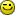). The maximum must be less than 20,000, but it's not clear how close we can get to that number. Is it possible to have a solution require over 10,000 steps? If you manage to create such a maze, please post in the comments!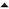01-'16 Facebook Hacker Cup 2016: Qualification Round problem analysis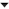01-'14 Fenwick trees demystified

An input-file for Cooking the Books with 100,000 digits can be found here.

Great explanations!
I always think its impressive that you write such short code!
C# is quite verbose and my 3rd assignment took over 180 lines of code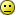Reader challenge: You might think that adding a timestamp creates four times as many states. In reality it only doubles the state space for this problem. Can you figure out why?

Although 4 states are supported, the cursor(current point) can only be at an EVEN or ODD state.
Example maze:
1 2 3 4
5 6 7 8

Stepping from 1 to 1 -> Step 1: 1, Step 2: 5, Step 3; 1.
Stepping from 1 to 1 -> step 1: 1, step 2: 2, step 3: 6, step 4: 5, step 5:1
It is impossible to get an 'even' step on 1.

Deducing: A point in the maze can only be countered after n-steps, which is either odd or even, but never both, thus only doubling in size.

[Comment edited on maandag 12 januari 2015 23:06]

ah. reading this, I realized my mistake in my solution for problem 2. My solution would have worked in O(2n), except that I thought that the state space was n2 so I terminate too early. Pretty dumb mistake.

When will the official answers to the problem inputs be posted? I had problems uploading the source code to problem 3, but I would like to see if it was correct. I had a lot of issues debugging my solution, because I do not know the tools of the trade for the language I chose (also C). Something to figure out before the next round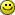A maze requiring 6794 steps: the file laser_maze_extreme_input.txt